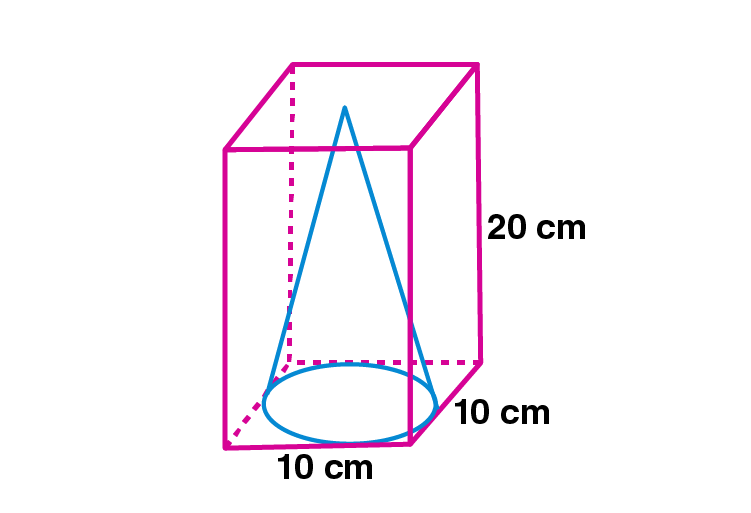Newbie

# A cone of maximum volume is curved out of a block of wood of size 20 cm x 10 cm x 10 cm. Find the volume of the remaining wood.

• 0

M.L Aggarwal book Important Question of class 10 chapter Based on Mensuration for ICSE BOARD.
A cone of maximum volume is curved out of a block of wood of given size. Find the volume of the remaining wood.
This is the Question Number 04, Exercise 17.4 of M.L Aggarwal.

Share

1.Given dimensions of the block of wood = 20 cm × 10 cm× 10 cm

Volume of the block of wood = 20×10×10 = 2000 cm3 [Volume = lbh]

Diameter of the cone, d = 10 cm

Radius of the cone , r = d/2 = 10/2 = 5 cm

Height of the cone, h = 20 cm

Volume of the cone = (1/3)r2h

= (1/3)×(22/7)×52×20

= (22×25×20)/(3×7)

= 11000/21 cm3

Volume of the remaining wood = Volume of block of wood – Volume of cone

= 2000-11000/21

= (21×2000-11000)/21

= (42000-11000)/21

= 31000/21

= 1476.19 cm3

Hence the volume of the remaining wood is 1476.19 cm3.

• 3